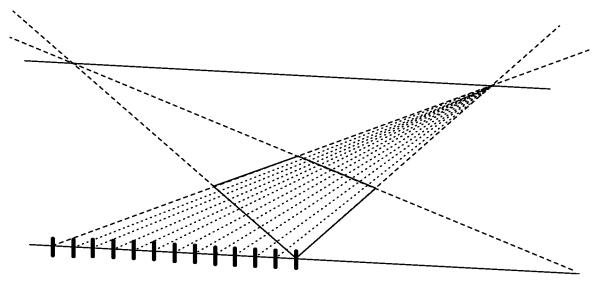## How do you draw a grid in two point perspective?

This question faced me recently, trying to predict coordinates for a grid in two point perspective. The problem is foreshortening. How do you figure out how much closer together rows become as a grid recedes into the distance?

A solution for one point perspective already exists on the web. I've also found a method for constructing a two point perspective grid, but limited to grids with 2^n number of squares.

Well, here's how to do it for any number of squares. I figured it out and I'm making this page so you can know how, too.

Now, when I approached this problem, I wanted to find a grid of points given that I knew the locations of the corners -- and nothing else. If you already have a horizon and vanishing points set up, you can skip ahead.

Anyway, first, the base. This is what I started with: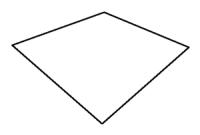The first thing to do is find the vanishing points. For any rectilinear object on a plane, there are two vanishing points. (Assuming that none of the sides is perfectly parallel to the horizon.)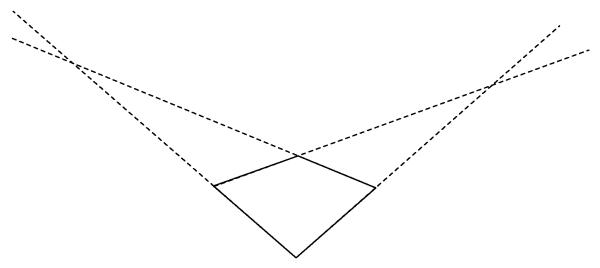Now we can define the horizon line. Since both vanishing points must lie on the horizon, then the horizon must be the line between those two points. (Note that horizons don't have to be horizontal -- imagine a poster on a wall, for example, viewed from the side. It has a horizon that is vertical.)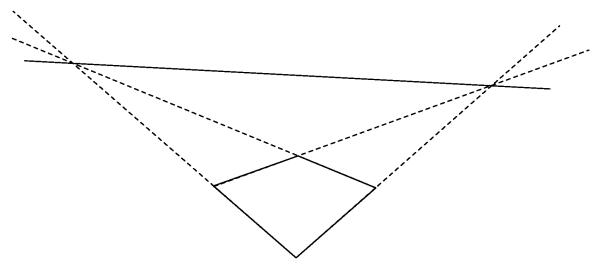Next, we draw a line parallel to the horizon, going through the bottommost point. Honestly, you could draw this line anywhere, as long as it isn't coincident with the horizon. It could even be above the horizon.Now let's find the projected length of a side. This is the length that side would be if you rotate the rectangle to make that side parallel to the horizon.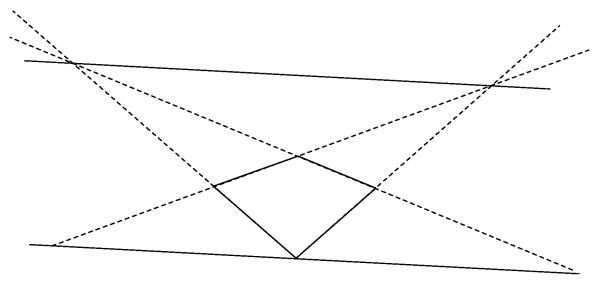Now we can subdivide that into however many regular units you wish for your grid. Here, I have 12 rows created. Because this line is parallel to the horizon, no foreshortening occurs on it! So a regular spacing on this line is a "true" regular spacing.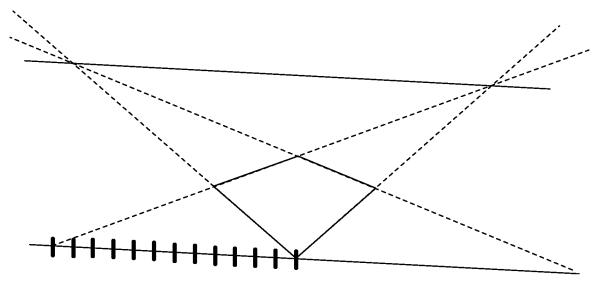Now just project each of those points along the foreground line back to your vanishing point. Those are the lines that define the grid! To get the perpendicular lines, of course, you just the same thing on the other side.